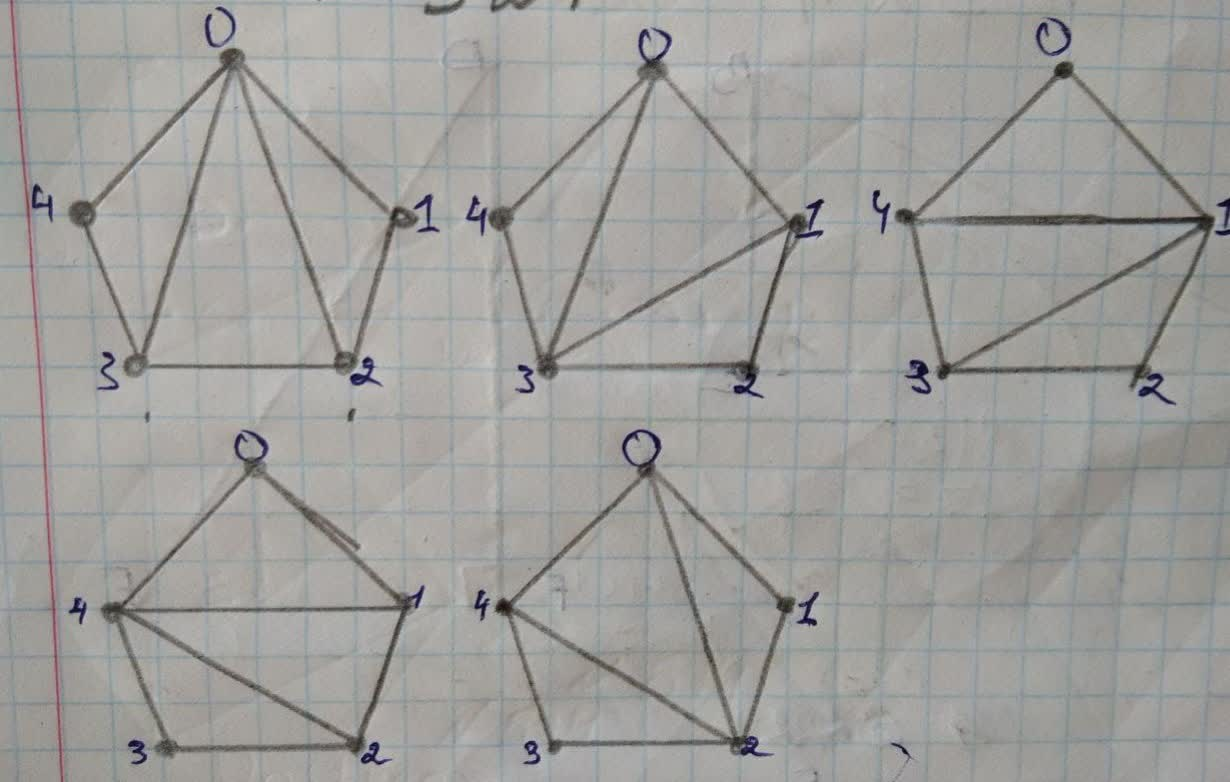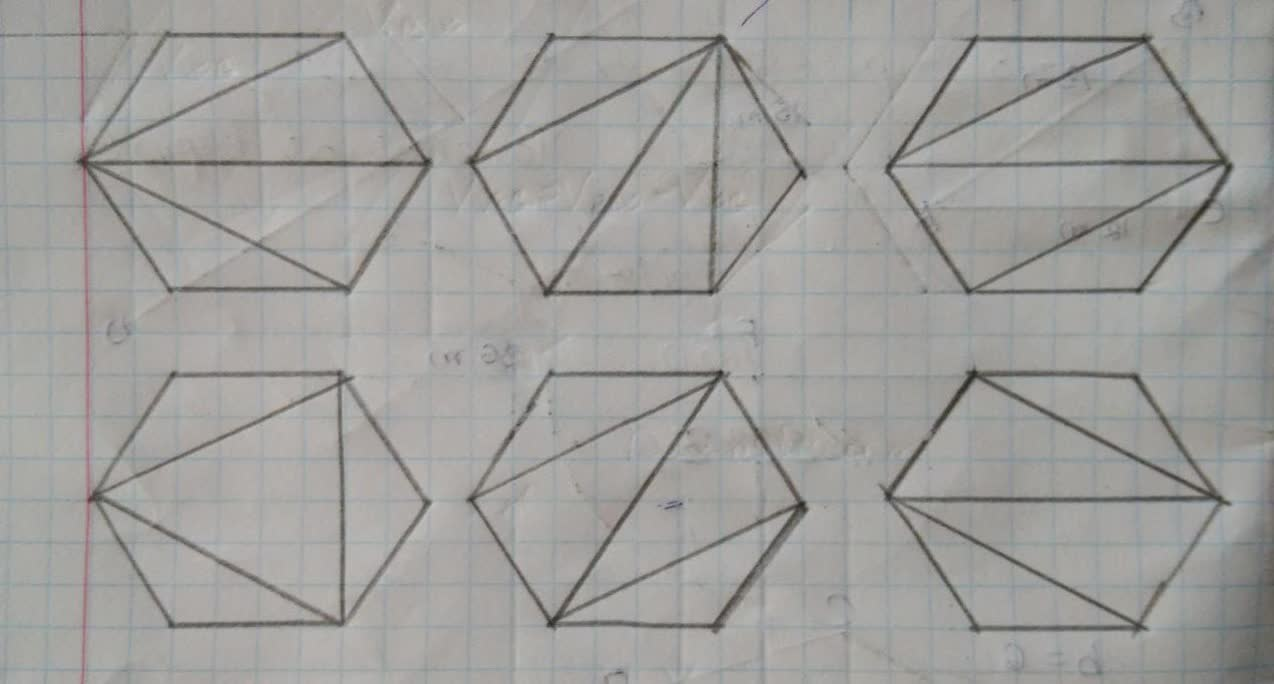## Look at the triangulation of convex polygons again. Prove following statements:

GeometryLook at the triangulation of convex polygons again. Prove following statements:8 months ago
Look at the triangulation of convex polygons again. Prove following statements:
Any triangulation of a convex n-polygon subdivides it into n - 2 triangles.
For example, every triangulation of a convex pentagon has 5 - 2=3 triangles, and every triangulation of a hexagon has 6-2=4 triangles
Step1 In the question we have to prove that any triangulation of a convex n-polygon subdivides it into n - 2 triangles. Step2 A convex polygon can be defined as a polygon whose interior angles are less than 180°.A triangulation of a convex polygon is given by drawing diagonals between non-adjacent vertices or we can say corners , also whose diagonal never intersect another diagonal (except at a vertex), until all possible ways of drawing diagonals have been used. For proof of triangulation of a convex n-polygon subdiides it into n- 2 triangles we will sketch polygon of 5 and 6 sides and show every possible diagonal. when n=5 as we can clearly see there are only 3 triangles possible in a convex pentagon, which proves thestatement any triangulation of a convex n-polygon subdivides it into n - 2 triangles. when n=6 as we can clearly see there are only 3 triangles possible in a convex hexagon, which proves the statement any triangulation of a convex n-polygon subdivides it into n - 2 triangles.